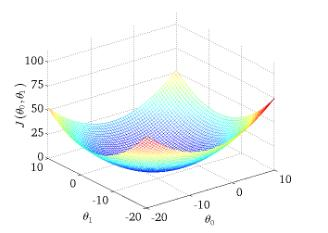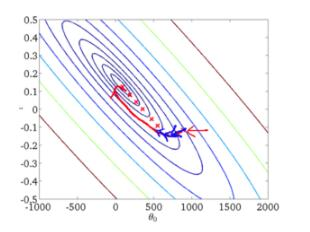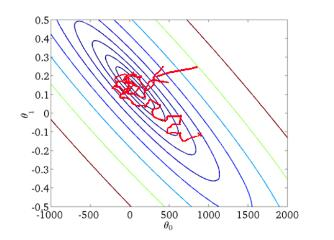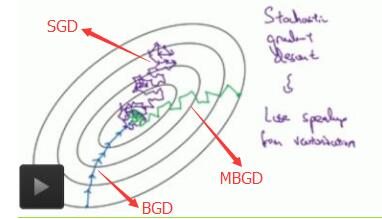# 批量梯度下降(BGD)、随机梯度下降(SGD)以及小批量梯度下降(MBGD)的理解

https://www.cnblogs.com/lliuye/p/9451903.html

为了便于理解，这里我们将使用只含有一个特征的线性回归来展开。此时线性回归的假设函数为：

hθ(x(i))=θ1x(i)+θ0hθ(x(i))=θ1x(i)+θ0

其中 i=1,2,...,mi=1,2,...,m 表示样本数。
对应的目标函数（代价函数）即为：

J(θ0,θ1)=12mi=1m(hθ(x(i))y(i))2J(θ0,θ1)=12m∑i=1m(hθ(x(i))−y(i))2

下图为 J(θ0,θ1)J(θ0,θ1) 与参数 θ0,θ1θ0,θ1 的关系的图：批量梯度下降法是最原始的形式，它是指在每一次迭代时使用所有样本来进行梯度的更新。从数学上理解如下：
（1）对目标函数求偏导：

ΔJ(θ0,θ1)Δθj=1mi=1m(hθ(x(i))y(i))x(i)jΔJ(θ0,θ1)Δθj=1m∑i=1m(hθ(x(i))−y(i))xj(i)

其中 i=1,2,...,mi=1,2,...,m 表示样本数， j=0,1j=0,1 表示特征数，这里我们使用了偏置项 x(i)0=1x0(i)=1 。
（2）每次迭代对参数进行更新：

θj:=θjα1mi=1m(hθ(x(i))y(i))x(i)jθj:=θj−α1m∑i=1m(hθ(x(i))−y(i))xj(i)

注意这里更新时存在一个求和函数，即为对所有样本进行计算处理，可与下文SGD法进行比较。
伪代码形式为：
repeat{
θj:=θjα1mmi=1(hθ(x(i))y(i))x(i)jθj:=θj−α1m∑i=1m(hθ(x(i))−y(i))xj(i)
(for j =0,1)
}

优点：
（1）一次迭代是对所有样本进行计算，此时利用矩阵进行操作，实现了并行。
（2）由全数据集确定的方向能够更好地代表样本总体，从而更准确地朝向极值所在的方向。当目标函数为凸函数时，BGD一定能够得到全局最优。
缺点：
（1）当样本数目 mm 很大时，每迭代一步都需要对所有样本计算，训练过程会很慢。
从迭代的次数上来看，BGD迭代的次数相对较少。其迭代的收敛曲线示意图可以表示如下：随机梯度下降法不同于批量梯度下降，随机梯度下降是每次迭代使用一个样本来对参数进行更新。使得训练速度加快。
对于一个样本的目标函数为：

J(i)(θ0,θ1)=12(hθ(x(i))y(i))2J(i)(θ0,θ1)=12(hθ(x(i))−y(i))2

（1）对目标函数求偏导：

ΔJ(i)(θ0,θ1)θj=(hθ(x(i))y(i))x(i)jΔJ(i)(θ0,θ1)θj=(hθ(x(i))−y(i))xj(i)

（2）参数更新：

θj:=θjα(hθ(x(i))y(i))x(i)jθj:=θj−α(hθ(x(i))−y(i))xj(i)

注意，这里不再有求和符号
伪代码形式为：
repeat{
for i=1,...,m{
θj:=θjα(hθ(x(i))y(i))x(i)jθj:=θj−α(hθ(x(i))−y(i))xj(i)
(for j =0,1)
}
}

优点：
（1）由于不是在全部训练数据上的损失函数，而是在每轮迭代中，随机优化某一条训练数据上的损失函数，这样每一轮参数的更新速度大大加快。
缺点：
（1）准确度下降。由于即使在目标函数为强凸函数的情况下，SGD仍旧无法做到线性收敛。
（2）可能会收敛到局部最优，由于单个样本并不能代表全体样本的趋势。
（3）不易于并行实现。

解释一下为什么SGD收敛速度比BGD要快：
答：这里我们假设有30W个样本，对于BGD而言，每次迭代需要计算30W个样本才能对参数进行一次更新，需要求得最小值可能需要多次迭代（假设这里是10）；而对于SGD，每次更新参数只需要一个样本，因此若使用这30W个样本进行参数更新，则参数会被更新（迭代）30W次，而这期间，SGD就能保证能够收敛到一个合适的最小值上了。也就是说，在收敛时，BGD计算了 10×30W10×30W 次，而SGD只计算了 1×30W1×30W 次。

从迭代的次数上来看，SGD迭代的次数较多，在解空间的搜索过程看起来很盲目。其迭代的收敛曲线示意图可以表示如下：小批量梯度下降，是对批量梯度下降以及随机梯度下降的一个折中办法。其思想是：每次迭代 使用 ** batch_size** 个样本来对参数进行更新。
这里我们假设 batchsize=10batchsize=10 ，样本数 m=1000m=1000 。
伪代码形式为：
repeat{
for i=1,11,21,31,...,991{
θj:=θjα110(i+9)k=i(hθ(x(k))y(k))x(k)jθj:=θj−α110∑k=i(i+9)(hθ(x(k))−y(k))xj(k)
(for j =0,1)
}
}

优点：
（1）通过矩阵运算，每次在一个batch上优化神经网络参数并不会比单个数据慢太多。
（2）每次使用一个batch可以大大减小收敛所需要的迭代次数，同时可以使收敛到的结果更加接近梯度下降的效果。(比如上例中的30W，设置batch_size=100时，需要迭代3000次，远小于SGD的30W次)
（3）可实现并行化。
缺点：
（1）batch_size的不当选择可能会带来一些问题。

batcha_size的选择带来的影响：
（1）在合理地范围内，增大batch_size的好处：
a. 内存利用率提高了，大矩阵乘法的并行化效率提高。
b. 跑完一次 epoch（全数据集）所需的迭代次数减少，对于相同数据量的处理速度进一步加快。
c. 在一定范围内，一般来说 Batch_Size 越大，其确定的下降方向越准，引起训练震荡越小。
（2）盲目增大batch_size的坏处：
a. 内存利用率提高了，但是内存容量可能撑不住了。
b. 跑完一次 epoch（全数据集）所需的迭代次数减少，要想达到相同的精度，其所花费的时间大大增加了，从而对参数的修正也就显得更加缓慢。
c. Batch_Size 增大到一定程度，其确定的下降方向已经基本不再变化。

下图显示了三种梯度下降算法的收敛过程：posted @ 2019-07-14 09:51  Alexander  阅读(2093)  评论(0编辑  收藏  举报